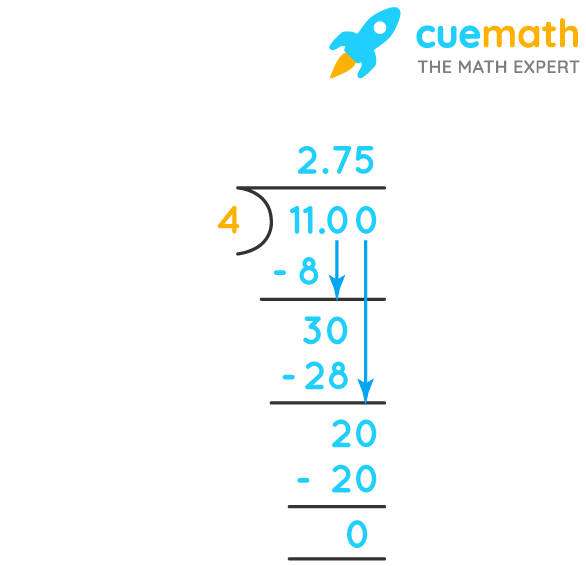# 2¾ As A Decimal

## Question: What is 2¾ as a decimal?

decimal number can be defined as a number whose whole number part and the fractional part is separated by a decimal point

## Answer: 2¾ can be written as 11/4 and it's decimal form is 2.75.

Let's convert 2¾ into decimal.

## Explanation:

2¾ can be written as 11/4

To convert any fraction to decimal form, we just need to divide its numerator by denominator.

The decimal form of 11/4 is given by dividing 11 by 4.